International
Tables for
Crystallography
Volume D
Physical properties of crystals
Edited by A. Authier

International Tables for Crystallography (2006). Vol. D, ch. 2.2, pp. 294-295

## Section 2.2.3. Symmetry operators

K. Schwarza*

aInstitut für Materialchemie, Technische Universität Wien, Getreidemarkt 9/165-TC, A-1060 Vienna, Austria
Correspondence e-mail: kschwarz@theochem.tuwein.ac.at

### 2.2.3. Symmetry operators

| top | pdf |

The concepts of symmetry operations in connection with a quantum-mechanical treatment of the electronic states are essential for an understanding of the electronic structure. In this context the reader is referred, for example, to the book by Altmann (1994).

For the definition of symmetry operators we use in the whole of this chapter the active picture, which has become the standard in solid-state physics. This means that the whole configuration space is rotated, reflected or translated, while the coordinate axes are kept fixed.

A translation is given by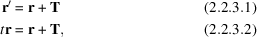where t on the left-hand side corresponds to a symmetry (configuration-space) operator.

#### 2.2.3.1. Transformation of functions

| top | pdf |

Often we are interested in a function (e.g. a wavefunction)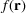and wish to know how it transforms under the configuration operator g which acts on. For this purpose it is useful to introduce a function-space operatorwhich defines how to modify the function in the transformed configuration space so that it agrees with the original functionat the original coordinate: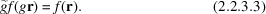This must be valid for all pointsand thus also for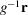, leading to the alternative formulation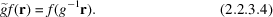The symmetry operations form a group G of configuration-space operationswith the related group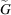of the function-shape operators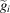. Since the multiplication rules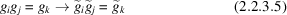are preserved, these two groups are isomorphic.

#### 2.2.3.2. Transformation of operators

| top | pdf |

In a quantum-mechanical treatment of the electronic states in a solid we have the following different entities: points in configuration space, functions defined at these points and (quantum-mechanical) operators acting on these functions. A symmetry operation transforms the points, the functions and the operators in a clearly defined way.

Consider an eigenvalue equation of operator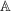(e.g. the Hamiltonian):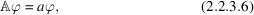whereis a function of. When g acts on, the function-space operatoracts [according to (2.2.3.4)] onyielding: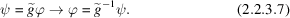By puttingfrom (2.2.3.7)into (2.2.3.6), we obtain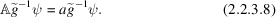Multiplication from the left byyields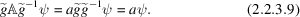This defines the transformed operator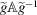which acts on the transformed functionthat is given by the original functionbut at position.

#### 2.2.3.3. The Seitz operators

| top | pdf |

The most general space-group operation is of the form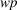with the point-group operation p (a rotation, reflection or inversion) followed by a translation w: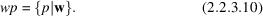With the definition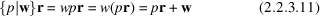it is easy to prove the multiplication rule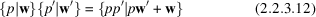and define the inverse of a Seitz operator as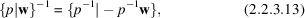which satisfies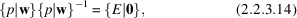where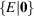does not change anything and thus is the identity of the space group G.

#### 2.2.3.4. The important groups and their first classification

| top | pdf |

Using the Seitz operators, we can classify the most important groups as we need them at the beginning of this chapter:

 (i) the space group, which consists of all elements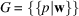; (ii) the point group (without any translations)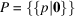; and (iii) the lattice translation subgroup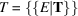, which is an invariant subgroup of G, i.e.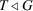. Furthermore T is an Abelian group, i.e. the operation of two translations commute (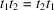) (see also Section 1.2.3.1of the present volume). A useful consequence of the commutation property is that T can be written as a direct product of the corresponding one-dimensional translations,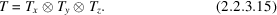(iv) A symmorphic space group contains no fractional translation vectors and thus P is a subgroup of G, i.e.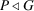. (v) In a non-symmorphic space group, however, some p are associated with fractional translation vectors. Thesedo not belong to the translation lattice but when they are repeated a specific integer number of times they give a vector of the lattice. In this case,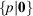can not belong to G for all p. (vi) The Schrödinger group is the group S of all operationsthat leave the Hamiltonian invariant, i.e.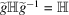for all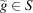. This is equivalent to the statement thatand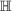commute: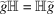. From this commutator relation we find the degenerate states in the Schrödinger equation, namely that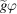andare degenerate with the eigenvalue E whenever, as follows from the three equations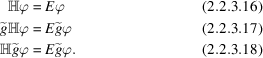### References

Altmann, S. L. (1994). Band theory of solids: An introduction from the view of symmetry. Oxford: Clarendon Press.